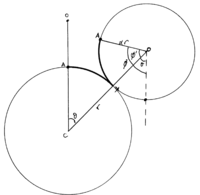# Revolutions of circles question

#### Dreyzo

##### New member
In this question, does it state that the starting point for Circle A is directly on the the edge of circle B ? Or is it actually on the edge of A? I am confused with the wording.

#### Attachments

• 1.1 MB Views: 14

#### pka

##### Elite Member
In this question, does it state that the starting point for Circle A is directly on the the edge of circle B ? Or is it actually on the edge of A? I am confused with the wording.
Suppose that $$R$$ is the radius of the large circle and $$r$$ is the radius of the small circle.
We are given that $$R=3\cdot r$$ WHY?
The circumference of the large circle is $$2\cdot R\cdot\pi~\&~$$ the circumference of the small circle is $$2\cdot r\cdot\pi~.$$
If the small circle "roles" around the large circle, what is the relation of the two circumferences?

#### Subhotosh Khan

##### Super Moderator
Staff member
In this question, does it state that the starting point for Circle A is directly on the the edge of circle B ? Or is it actually on the edge of A? I am confused with the wording.
The answer to this question is not "intuitive". Please watch:

•jonah2.0

#### Jomo

##### Elite Member
I've seen this problem on this site before. One might assume the answer is 3, but try again!

#### lex

##### Full Member
To convince myself of the solution, I compared angular speeds.When the line joining the centres, CO, has turned through an angle $$\displaystyle \theta$$ radians, the radius line of the 'small' circle, OA, has turned through $$\displaystyle \boxed{\; \phi = \phi' + \theta \;}$$ radians (from the original downward vertical direction), and the arc lengths AX on the two circles are equal (because rolling).

This last fact means that:
\displaystyle \begin{align*} \hspace25ex r\theta &= \alpha r \phi'\\ \phi'&=\tfrac{1}{\alpha}\theta\\ \therefore \phi&=\left(1+\tfrac{1}{\alpha}\right)\theta \end{align*}

The ratio of the angular speeds is $$\displaystyle 1+\tfrac{1}{\alpha}$$

\displaystyle \begin{align*} \text{In the example originally posted, } \;\alpha&=\tfrac{1}{3}\\ \therefore \phi&=4\theta \end{align*}
The 'small' circle makes 4 complete turns as $$\displaystyle \theta$$ maps out 1 complete turn.

Another way of looking at the motion is to keep A in contact with the big circle and slide the small circle (don't roll it) down the big circle, so that A lands at X. By this sliding OA is already $$\displaystyle \theta$$ radians from the original downward vertical direction. Now rotate the small circle (without rolling) to give the current picture. OA turns a further $$\displaystyle \phi'$$ radians.

Last edited:
•Subhotosh Khan

#### lex

##### Full Member
This last point gives a nice way of thinking of the problem.
When the small circle Rolls a certain distance around the large circle, the point of contact on the small circle 'slides' along the circumference of the big circle and the small circle rotates about its own centre. For the purpose of the question, these simultaneous motions can be considered separately:
(a) first sliding the original point of contact A along the circumference of the big circle a certain distance, and then
(b) rotating the small circle about its centre, so that the point A moves a distance equal to the distance it has slid on the circumference of the big circle.
(a) The sliding turns OA through $$\displaystyle \boldsymbol{\theta}$$ radians, and
(b) the rotating of the small circle about its own centre, turns OA a further $$\displaystyle \phi'=\boldsymbol{\tfrac{1}{\alpha}\theta}\;$$ radians.

So in rolling the whole way around the big circle, the motion can be broken into:
(a) the small circle sliding (point A) a complete turn of the big circle, so OA turns through 1 complete turn
(b) and then the small circle rotating about its centre so that A moves a distance equal to the distance it has slid i.e. the circumference of the big circle, which requires 3 complete turns of the small circle.
In total OA makes 4 complete turns.

•Subhotosh Khan